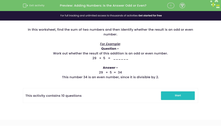### Comprehensive & curriculum aligned

In this worksheet, students add together two numbers and decide whether the answer is an odd or even number.Key stage:  KS 2

Curriculum topic:   Number: Addition and Subtraction

Popular topics:   Subtraction worksheets, Addition worksheets

Difficulty level:#### Worksheet Overview

In this worksheet, find the sum of two numbers and then identify whether the result is an odd or even number.

For Example:

Question -
Work out whether the result of this addition is an odd or even number.

29   +  5   =   ______

29   +  5  =  34

This number 34 is an even number, since it is divisible by 2.

### What is EdPlace?

We're your National Curriculum aligned online education content provider helping each child succeed in English, maths and science from year 1 to GCSE. With an EdPlace account you’ll be able to track and measure progress, helping each child achieve their best. We build confidence and attainment by personalising each child’s learning at a level that suits them.

Get started